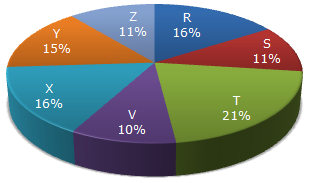# Data Interpretation - Pie Charts - Discussion

Study the following pie-chart and the table and answer the questions based on them.

Proportion of Population of Seven Villages in 1997Village % Population Below Poverty Line X 38 Y 52 Z 42 R 51 S 49 T 46 V 58

2.

The ratio of population of village T below poverty line to that of village Z below poverty line in 1997 is:

 [A]. 11 : 23 [B]. 13 : 11 [C]. 23 : 11 [D]. 11 : 13

Explanation:

Let N be the total population of all the seven villages.

Then, population of village T below poverty line = 46% of (21% of N)

and Population of villages Z below the poverty line = 42% of (11% of N)Required ratio = 46% of (21% of N) = 46 x 21 = 23/11. 42% of (11 % of N) 42 x 11

 Praveen said: (Jan 10, 2011) How this possible explain briefly please?

 Ravi said: (Dec 11, 2011) @Praveen The total population has to consider for calculating the ratios.. And so 46*21 / 42*11 would give u the answer 23:11

 Divya said: (Jun 3, 2014) How 46*21 and 42*11 came. What values are 21 and 11?

 Gupreet Kaur said: (Jul 1, 2014) What value are 21 and 11? how this arranged? please any one explain me.

 Mantu Kumar Deka said: (May 19, 2015) Don't know how the value of 21 and 11 came? Any one can help on this?

 Phub said: (Jul 12, 2015) The value of 21 and 11 is the value of t and z from the above pie chart.

 Sushmitha said: (Apr 27, 2018) Why they have taken N in this problem?

 Ravindhiran said: (Jul 26, 2018) T = 21% ( in 21% of population 46% people are below powerty line). Z= 11 % ( in 11% of T population, 42% people are below P line). = 46% of 21/42% of 11. = 46*21/42*11. = 23/11.

 Dorji said: (Sep 22, 2018) Could not get the concept. Anyone help me by explaining it.

 Divyavishnu said: (Dec 17, 2018) Anyone help me by explaining it.

 Namgay Zangpo said: (Jun 30, 2019) 46*21=966/42*11=462. But how are we getting answer 23/11?

 Tshering Yangchen said: (Sep 20, 2020) Can anyone help me to solve this question?

 Kezang said: (Oct 5, 2020) 42 * 23 = 966. 42 * 11 = 462.